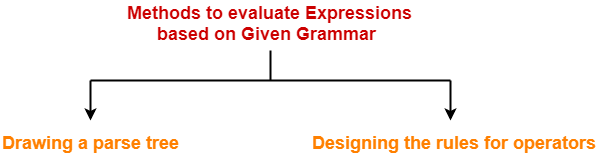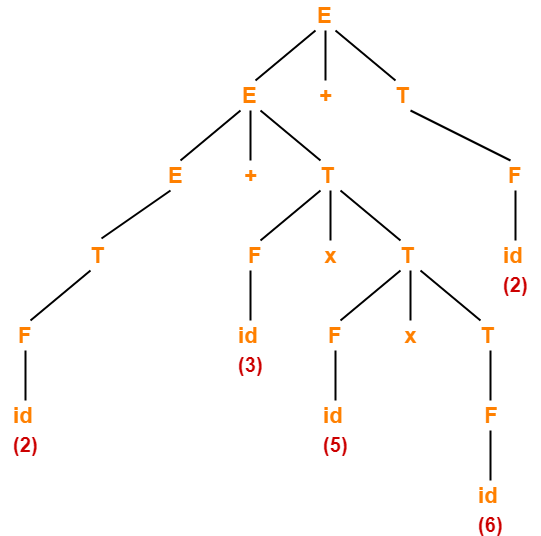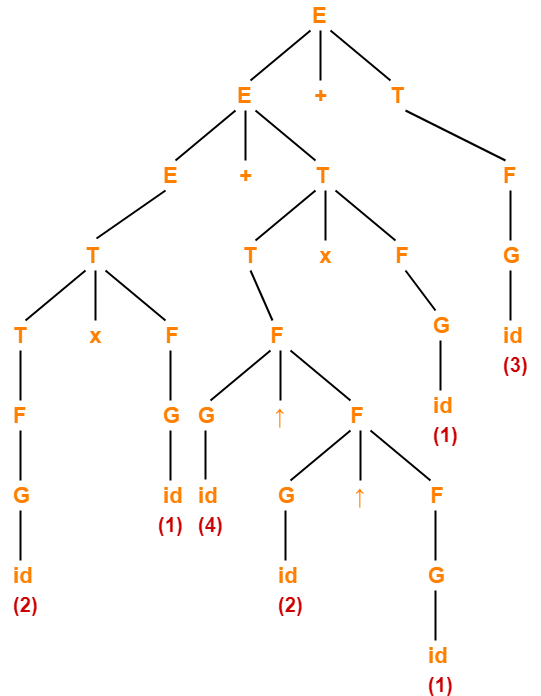# Evaluating Expressions Based On Given Grammar

## Evaluating Expressions Based On Given Grammar-

There are two methods for evaluating the expressions based on given grammar-1. Drawing a parse tree
2. Designing the rules for operators

### Method-01: Drawing Parse Tree-

In this method, following steps are followed-

• We draw a Parse Tree for the given expression.
• We evaluate the parse tree to get the required value of the expression.

### Drawback-

• Drawing a parse tree for large expressions is cumbersome and time taking.
• So, this method becomes difficult to follow when the expression is large.

### Method-02: Designing Rules For Operators-

In this method, following steps are followed-

• We decide the priority and associativity of operators in the grammar.
• We parenthesize the given expression.
• We evaluate the expression to get the required value.

• This method is easy to follow whether the expression is small or large.
• So, this method is preferred.

### Rules For Deciding Priority Of Operators-

For the given unambiguous grammar,

• The priority of operators is decided by checking the level at which the production is present.
• The higher the level of production, the lower the priority of operator contained in it.
• The lower the level of production, the higher the priority of operator contained in it.

### Example-

Consider the grammar-

E → E x F / F + E / F

F → F – T / T

T → id

Here,

• id has the highest priority.

(since T → id is present at the bottom most level)

• x and + operators have the least priority.

(since E → E x F / F + E are present at the top most level)

• x and + operators have the same priority.

(since E → E x F / F + E are present at the same level)

• – operator has higher priority than x and + but lesser priority than id.

(since F → F – T is present at the middle level)

So, the priority order is-

id > > ( x , + )

### Rules For Deciding Associativity Of Operators-

For the given unambiguous grammar,

• The associativity of operators is decided by checking the Type Of Recursion in the production.
• If the production has left recursion, then the operator is left associative.
• If the production has right recursion, then the operator is right associative.
• If the production has both left and right recursion, then the operator is neither left associative nor right associative.

### Example-

Consider the grammar-

E → E x F / F + E / F

F → F – F / T

T → id

Here,

• x operator is left associative.

(since E → E x F has left recursion in it)

• + operator is right associative.

(since E → F + E has right recursion in it)

• F – F is neither left associative nor right associative.

(since F → F – F has both left and right recursion in it)

## Problem-01:

Consider the given grammar-

E → E + T / T

T → F x T / F

F → id

Evaluate the following expression in accordance with the given grammar-

2 + 3 x 5 x 6 + 2

## Solution-

### Method-01:

• Let us draw a parse tree for the given expression.
• Evaluating the parse tree will return the required value of the expression.

The parse tree for the given expression is-On evaluating this parse tree, we get the value = 94.

### Method-02:

The priority order and associativity of operators on the basis of given grammar is-

id > x > +

where-

• x operator is right associative.
• + operator is left associative.

Now, we parenthesize the given expression based on the precedence and associativity of operators as-

( 2 + ( 3 x ( 5 x 6 ) ) ) + 2

Now, we evaluate the parenthesized expression as-

= ( 2 + ( 3 x ( 5 x 6 ) ) ) + 2

= ( 2 + ( 3 x 30 ) ) + 2

= ( 2 + 90 ) + 2

= 92 + 2

= 94

## Problem-02:

Consider the given grammar-

E → E + T / E – T / T

T → T x F / T ÷ F / F

F → G ↑ F / G

G → id

Evaluate the following expression in accordance with the given grammar-

2 x 1 + 4 ↑ 2 ↑ 1 x 1 + 3

## Solution-

### Method-01:

• Let us draw a parse tree for the given expression.
• Evaluating the parse tree will return the required value of the expression.

The parse tree for the given expression is-On evaluating this parse tree, we get the value = 21.

### Method-02:

The priority order and associativity of operators on the basis of given grammar is-

id > > ( x , ÷ ) > ( + , )

where-

• + , – , x , ÷ operators are left associative.
• ↑ operator is right associative

Now, we parenthesize the given expression based on the precedence and associativity of operators as-

( ( 2 x 1 ) + ( ( 4 ↑ ( 2 ↑ 1 ) ) x 1 ) ) + 3

Now, we evaluate the parenthesized expression as-

= ( ( 2 x 1 ) + ( ( 4 ↑ ( 2 ↑ 1 ) ) x 1 ) ) + 3

= ( ( 2 x 1 ) + ( ( 4 ↑ 2 ) x 1 ) ) + 3

= ( ( 2 x 1 ) + ( 16 x 1 ) ) + 3

= ( 2 + ( 16 x 1 ) ) + 3

= ( 2 + 16 ) + 3

= 18 + 3

= 21

## Problem-03:

Consider the given grammar-

E → E + T / E – T / T

T → T x F / T ÷ F / F

F → F ↑ G / G

G → id

Evaluate the following expression in accordance with the given grammar-

2 ↑ 1 ↑ 4 + 3 x 5 x 6 ↑ 1 + 2 ↑ 3

## Solution-

The priority order and associativity of operators on the basis of given grammar is-

id > > ( x , ÷ ) > ( + , )

where + , – , x , ÷, ↑ operators are left associative.

Now, we parenthesize the given expression based on the precedence and associativity of operators as-

( ( ( 2 ↑ 1 ) ↑ 4 ) + ( ( 3 x 5 ) x ( 6 ↑ 1 ) ) ) + ( 2 ↑ 3 )

Now, we evaluate the parenthesized expression as-

= ( ( ( 2 ↑ 1 ) ↑ 4 ) + ( ( 3 x 5 ) x ( 6 ↑ 1 ) ) ) + ( 2 ↑ 3 )

= ( ( 2 ↑ 4 ) + ( ( 3 x 5 ) x ( 6 ↑ 1 ) ) ) + ( 2 ↑ 3 )

= ( 16 + ( ( 3 x 5 ) x ( 6 ↑ 1 ) ) ) + ( 2 ↑ 3 )

= ( 16 + ( ( 3 x 5 ) x 6 ) ) + ( 2 ↑ 3 )

= ( 16 + ( ( 3 x 5 ) x 6 ) ) + 8

= ( 16 + ( 15 x 6 ) ) + 8

= ( 16 + 90 ) + 8

= 106 + 8

= 114

## Problem-04:

Consider the given grammar-

E → E ↑ T / T

T → T + F / F

F → G – F / G

G → id

Evaluate the following expression in accordance with the given grammar-

2 ↑ 1 ↑ 3 + 5 – 6 – 8 – 5 + 10 + 11 ↑ 2

## Solution-

The priority order and associativity of operators on the basis of given grammar is-

id > > + >

where-

• + , ↑ operators are left associative.
• – is right associative.

Now, we parenthesize the given expression based on the precedence and associativity of operators as-

( ( 2 ↑ 1 ) ↑ ( ( ( 3 + ( 5 – ( 6 – ( 8 – 5 ) ) ) ) + 10 ) + 11 ) ) ↑ 2

Now, we evaluate the parenthesized expression as-

= ( ( 2 ↑ 1 ) ↑ ( ( ( 3 + ( 5 – ( 6 – ( 8 – 5 ) ) ) ) + 10 ) + 11 ) ) ↑ 2

= ( ( 2 ↑ 1 ) ↑ ( ( ( 3 + ( 5 – ( 6 – 3 ) ) ) + 10 ) + 11 ) ) ↑ 2

= ( ( 2 ↑ 1 ) ↑ ( ( ( 3 + ( 5 – 3 ) ) + 10 ) + 11 ) ) ↑ 2

= ( ( 2 ↑ 1 ) ↑ ( ( ( 3 + 2 ) + 10 ) + 11 ) ) ↑ 2

= ( ( 2 ↑ 1 ) ↑ ( ( 5 + 10 ) + 11 ) ) ↑ 2

= ( ( 2 ↑ 1 ) ↑ ( 15 + 11 ) ) ↑ 2

= ( ( 2 ↑ 1 ) ↑ 26 ) ↑ 2

= ( 2 ↑ 26 ) ↑ 2

= (226) ↑ 2

= (226)2

## Problem-05:

Consider the given priority order of operators-

id > ( x , ÷ ) > ( + , )

Given all the operators are left associative, construct the corresponding grammar.

## Solution-

We apply the rules learnt above in reverse direction to obtain the required grammar.

The corresponding grammar is-

E → E + T / E – T / T

T → T x F / T ÷ F / F

F → id

## Problem-06:

Consider the given priority order of operators-

id > > ( x , ÷ ) > ( + , )

Given all the operators are left associative, construct the corresponding grammar.

## Solution-

The corresponding grammar is-

E → E + T / E – T / T

T → T x F / T ÷ F / F

F → G ↑ F / G

G → id

Next Article- Important Points For Exams

Get more notes and other study material of Theory of Automata and Computation.

Watch video lectures by visiting our YouTube channel LearnVidFun.

SummaryArticle Name
Evaluating Expressions Based On Given Grammar
Description
Two methods for evaluating the expressions based on given grammar- Drawing a parse tree and Designing rules for operators. Rules for deciding priority and associativity of operators are given.
Author
Publisher Name
Gate Vidyalay
Publisher Logo